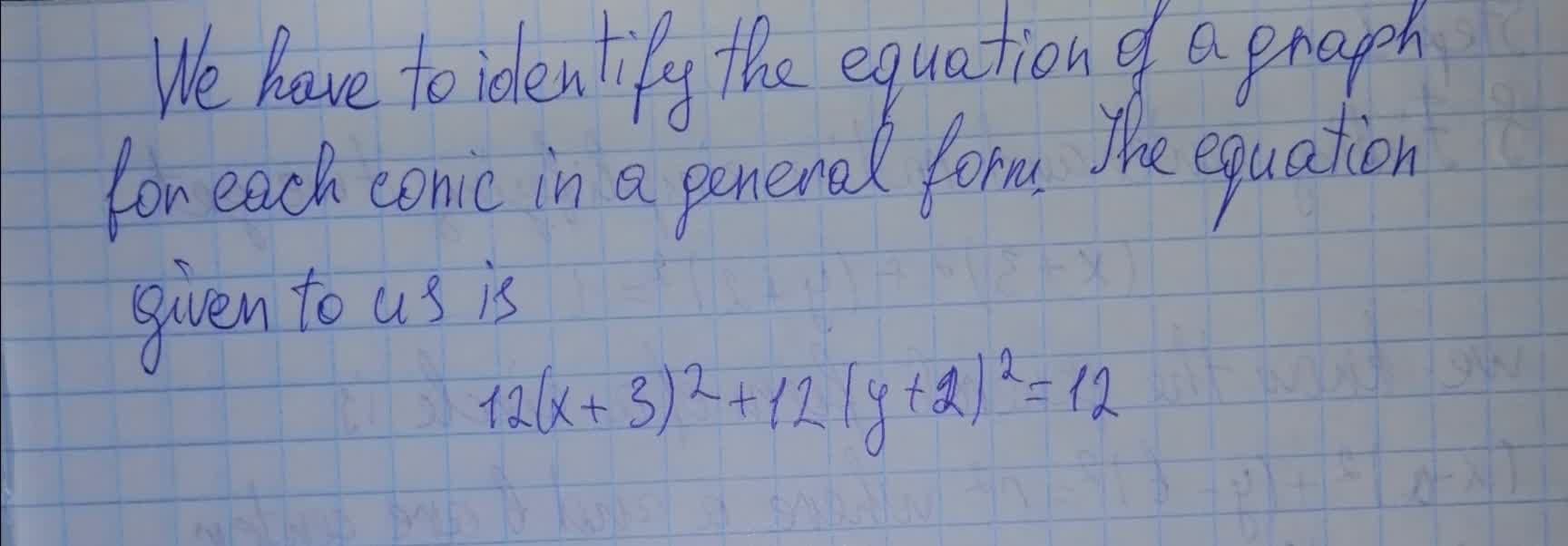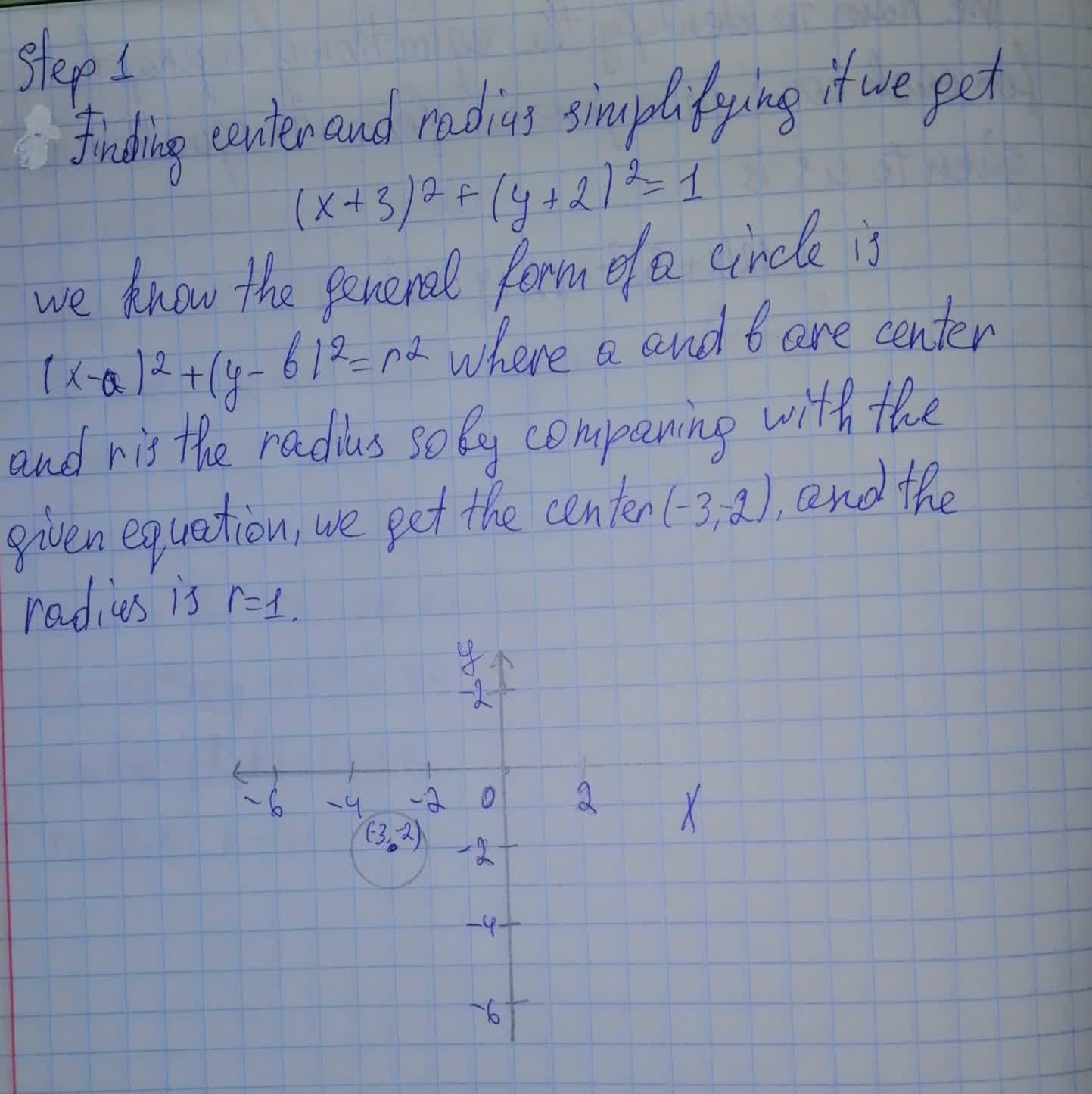Question# For hyperbola 12(x + 3)^2 + 12(y + 2)^2 = 12, find the asymptotes.

Conic sections
ANSWEREDFind the equation of the graph for each conic in general form. Identify the conic, the center, the vertex, the co-vertex, the focus (foci), major axis, minor axis, a 2, b 2, and c 2. For hyperbola, find the asymptotes. Sketch the graph. $$\displaystyle{12}{\left({x}+{3}\right)}^{{2}}+{12}{\left({y}+{2}\right)}^{{2}}={12}$$